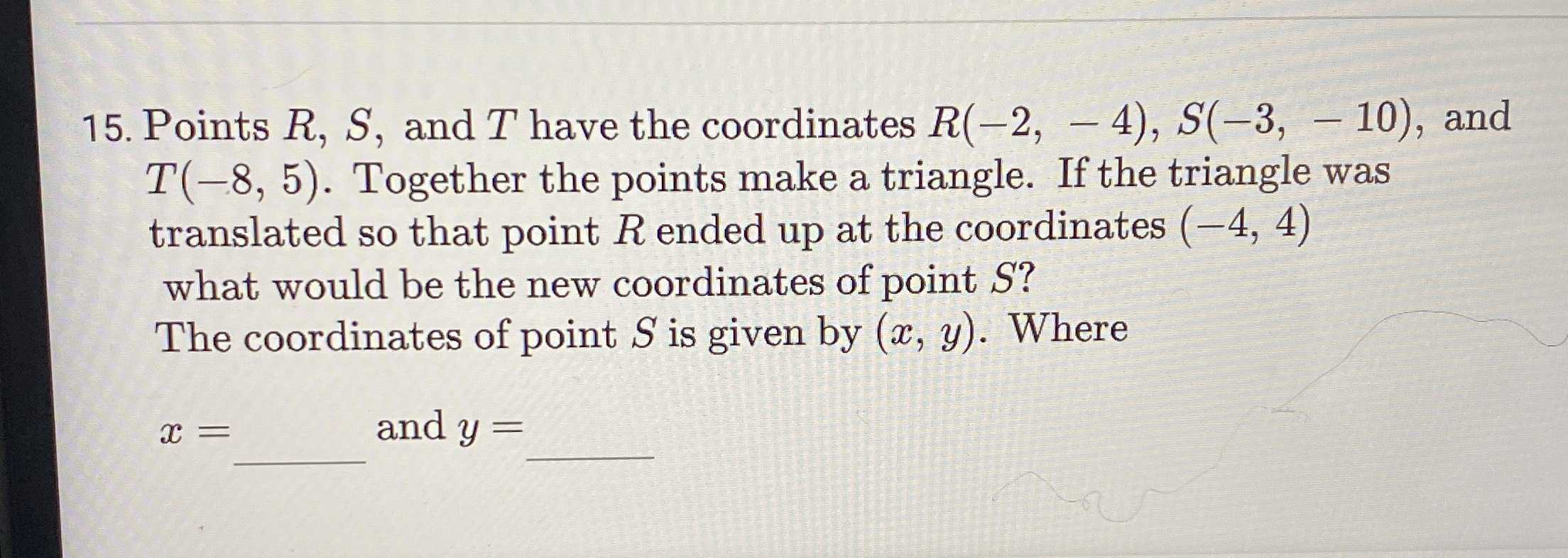### ¿Todavía tienes preguntas de matemáticas?

Pregunte a nuestros tutores expertos
Algebra
Pregunta15. Points $$R , S ,$$ and $$T$$ have the coordinates $$R ( - 2 , - 4 ) , S ( - 3 , - 10 )$$ , and

$$T ( - 8,5 )$$ . Together the points make a triangle. If the triangle was translated so that point $$R$$ ended up at the coordinates $$( - 4,4 )$$ what would be the new coordinates of point $$S ?$$ The coordinates of point $$S$$ is given by $$( x , y )$$ . Where

$$x = \quad$$ and $$y =$$